# VIDEO: Brief Review: Adding and Subtracting Rational Numbers

Watch this video about rational numbers. (The transcript is included.)

Video Transcript

In your study of integers you saw that dividing integers sometimes resulted in a non-integer quotient. In this video we look at a class of numbers called rational numbers, which also includes the integers.

Use the illustration to determine the ratio of boys to girls in the class, the fraction of boys in the class, and the fraction of girls in the class. Display the results as rational numbers.

A rational number is the ratio of two integers a and b, where b is not zero. The simple reason that b can’t be zero is that division by zero is undefined.

As written, a rational number looks like a fraction or a ratio, but integers can also be rational numbers. We’ll see examples of those later in the video.

In this example, we are given a classroom of boys and girls. The first task is to find the ratio of boys to girls.

Count the number of boys. As you can see there are 1-2-3-4-5-6-7 boys. Now count the number of girls. There are 1-2-3-4-5-6-7-8-9 girls. So the ratio of boys to girls is shown here. The ratio is 7/9 or 7 to 9. This is a rational number, since it meets the definition of a ratio of two integers, here and here.

Now we need to calculate the fraction of boys in the classroom. This is a different ratio. We know the number of boys is 7, now we need to find the total number of students. We can add these two numbers, 7 + 9, to get 16. Or we could see that the array here is 2 by 8, which also gives us 16.

So the fraction of boys is 7/16. This is also a rational number.

Finally, the fraction of girls is 9/16, also a rational number.

There are a couple more observations to make. First, the total number of students, the whole, is made up of two parts, the number of boys and the number of girls. So the ratio of boys to girls, this ratio, is a part to part ratio. The fraction of boys and girls are part-whole ratios. All of these are rational numbers, but some are fractional amounts.

Let’s look at another example.

On the number line, each tic mark represents a quarter inch. Describe the positions of the fish and the dragonfly using rational numbers.

With this vertical number line, the water line is the zero mark, as shown here. We are told that each tic mark represents a quarter.

Let’s start with the dragonfly and find its position. Starting at zero there are 1-2-3-4-5 tic marks, so the dragonfly is at position 5/4, as shown here. This is also equivalent to 1 ¼.

Now let’s find the position of the fish. Starting at zero, there are 1-2 tic marks. Since this position is below the zero mark, the number is negative. So the fish is at position -2/4, which is also equal to -½.

We rewrite the positions on the number line as a mixed number here and a fraction in simplest form here. These are rational numbers because they meet the definition of a rational number. Even the mixed number can be rewritten as an improper fraction.

Let’s look at a final example.

On a winter day in a given city the low and high temperatures for the day are shown in this double bar graph. Describe these temperatures relative to 0°F. Are these temperatures rational numbers?

Another way to express rational numbers are as decimals. The data shown in this graph shows values to the tenths place. However, not all values written in decimal form are rational numbers. For example, π is not a rational number.

The horizontal line represents 0°F. So, this bar represents a temperature of 25.8° above zero, and this temperature represents -10.5 degrees below zero.

How do we know that these decimals are rational numbers? Going back to the definition of a rational number, 25.8 can be rewritten as 258 over 10, which in simplest form is 129 over 5.

Likewise, -10.5 can be rewritten as -105 over 10, which simplifies to -21 over 2. When a decimal can be written as a ratio of two integers, with a non-zero term in the denominator, then the decimal is a rational number.

## What Are Rational Numbers?

### A Brief Review of Integers

Integers include the whole numbers, zero, and

positive numbers 1, 2, 3, etc., but also includes a different class of numbers, negative numbers.You can use a number line to represent the integers.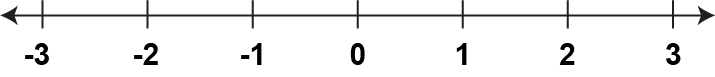Notice that every integer and its opposite is the same distance from 0 on the number line. Also, the arrow heads on the number line mean that the integers extend to infinity.

### Rational Numbers

All rational numbers can be written this way.Rational numbers can be written in different ways.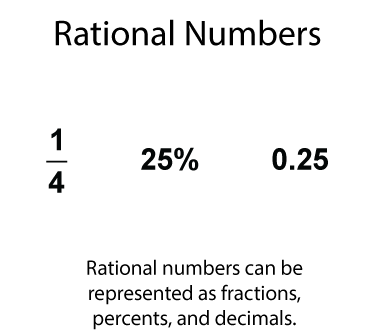Integers are examples of rational numbers.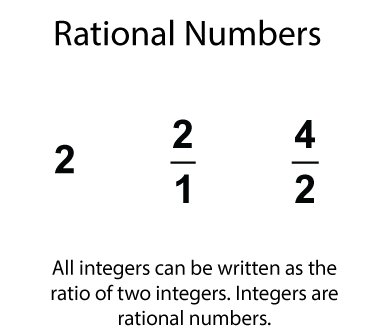If a number cannot be written as the ratio of two integers, then it is an irrational number.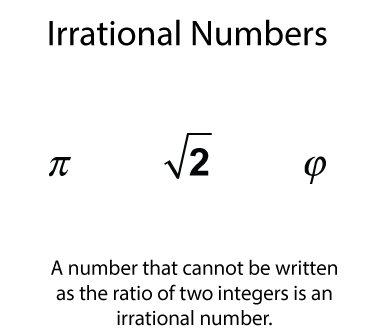Rational numbers can be graphed on a number line.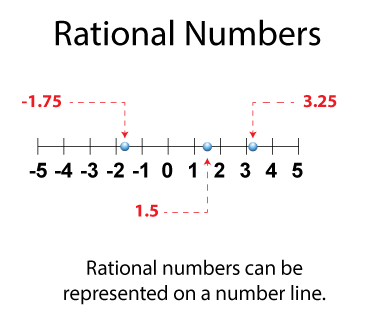In this Brief Review, the addition and subtraction of rational numbers is explored.

## Video Transcripts

### This video has a transcript available. To see the complete collection of video transcripts, click on this link.

Common Core Standards CCSS.MATH.CONTENT.7.NS.A.2.A, CCSS.MATH.CONTENT.8.NS.A.1, CCSS.MATH.CONTENT.7.NS.A.3 1.00 minutes 6 - 8 Algebra     • The Language of Math         • Numerical Expressions 2014 algebra, adding rational numbers, subtracting rational numbers